Visitors Online: 77 | Wednesday 16th October 2019
 CBSE Guess > Papers > Question Papers > Class XII > 2005 > Maths > Delhi Set-II MATHEMATICS 2005 (Set II—Delhi)
Except for the following questions, all the remaining questions have been asked in Set I. SECTION - A  Q .1 Four for the drawn randomly form a well shuffled pack of 52 cards. Find the probability of getting 3 diamonds and one spade. Q. 6. If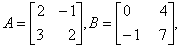find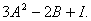Q. 9. If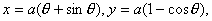findQ. 15. Prove that the line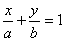is a tangent to the curve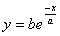at point where the curve cuts y-axis. Q. 17. Prove that the surface area of a solid cuboid, base and given volume, is minimum when it is a cube. SECTION - B  Q. 20. Forceand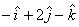act at a point P, with position vector. Find the vectormoment of the resultant of these forces about the point Q whose position vector isQ. 22. A car has a velocity of 30 m/sec at a certain instant. After 10 seconds, the velocity becomes 60 m/sec. If rate of change of velocity is uniform, find the distance this period. Q. 24. Show that the lines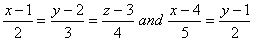intersect, also find he point of intersection. SECTION - C  Q. 20. An insurance company insured 2000 scooters and 3000 motor cycles. The probability of an accident involving a scooter is 0.01 and that of a motor cycle is 0.02. An insured vehicle met with an accident. Find the probability that the accidented vehicle was a motor cycle. Q. 24. A person deposits Rs.1,00,000 in a bank at the end of each year for 10 years. If the compound interest at 10% per annum is reckoned, that would be the sum standing to his credit at the end of the period? [Use (1.1) 10 =2.594] Maths 2005 Question Papers Class XII Delhi Outside Delhi Compartment Delhi Compartment Outside Delhi Set I Set I Set I Set I Set II Set II Set II Set II Set III Set III CBSE 2005 Question Papers Class XII